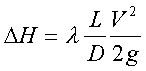The head loss represent pressure losses, (or load losses) in the conduct of air systems, Hydraulic or other fluids.

Software interfaces for calculating major and minor head loss

The head loss evolve with the fluid velocity, at low fluid velocities, the head loss are low and at high speeds the pressure losses are high.

The major pressure drop (major head loss) represent energy losses due to friction of the fluid in a conduit of constant section. They are expressed in fluid heights (in meters) and pascals.DH is the loss of load, in meters fluid column

V is the average flow velocity in meters per second

D is the diameter of the flow, in meters

L is the flow (pipe) length, in meters

l Is the Major head loss coefficient (unitless number) the coefficient of head loss is determined according to the flow regime

The equations used in the mecaflux software for the calculation of major head loss are:

calculation procedure with

Software for calculating head loss mecaflux Standard

To calculate the energy losses, certain information is required, enter the information in the "Parameters area"
Press calculate.. (if a required parameter is missing a message "insufficient parameters will warn) Pendant le calcul:
• You may be offered a choice of two equations, the results are similar, but you can compare different methods of calculation.
• You may be offered a choice between two flow regimes when you are close to the limits of transition between laminar and turbulent flow.. This transition is often brought into reality by defects in roughness. (if you restart the calculation by slightly modifying the roughness you switch to a regime or another)
Calculation results are displayed in the software's results area":
The inventory of head loss, allows you to add the results of several calculations, perform test debits, research points operating pumps and graphical analysis: See inventory pressure drop

Minor head loss calculation: The minor head loss are pressure losses caused by the passage of the fluid in obstacles such as valves, fittings, enlargement, reservoir outlet. They are expressed in Pascals or columns fluid meters (m). They are treated in head loss tab , according to the element's parameters you choose.
• Procedure for calculating minor pressure drop with mecaflux standard
• select an minor loss element by choosing a "category" and select "element"
• fill in the fields with blue text in "Parameters" area
• click calculate
If a text box has not been entered correctly a message will tell you. The results are given in meters colomn fluid and pascals.They are added in the inventory load losses to study all losses in a network. See inventory pressure drop